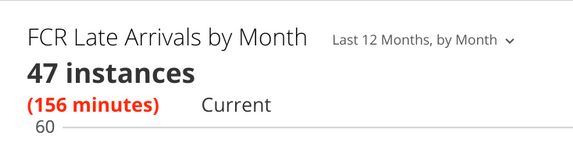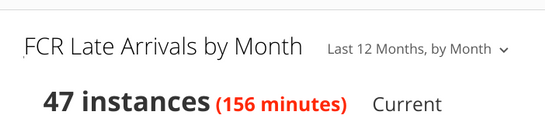# HTML/CSS issue (Summary# data showing on separate lines)

Did a lot of reading from previous posts about using HTML/CSS in Summary#'s and wanted to give it a try for a card, and while it looks great and provides a very effective quick view to determine lost time, can't seem to get this to display properly, i.e. on a single line (we wanted to make the lost time standout, using RED as the color).

Just wondering if another pair of eyes might be able to pickup on the issue in the HTML/CSS markup we're using that places the two metrics onto separate lines within the Summary# calculation? I'm pretty certain it has to do with the DIV's being used, possibly the placement, but can't seem to find another way to show the total time in RED without placing that within a second DIV which seems to be the culprit?

Any thoughts on updates to the markup for this? Thanks!

SUMMARY NUMBER:

CONCAT (

'<div style="font-size: 28px; width: 100%; height:100%; margin:-20px; padding:20px;">', CONCAT

(COUNT(CASE WHEN `Late By` <> '' THEN (SUBSTRING(`Late By`, 1, (INSTR(`Late By`, 'm')-1)) ) + SUBSTRING(`Late By`, (INSTR(`Late By`, 'm')+2), (INSTR(`Late By`, 's'))-(INSTR(`Late By`, 'm')+2)) / 60 ELSE 0 END), ' instances','

<div style="font-size: 20px; color: red;">

(', SUM(CASE WHEN `Late By` <> '' THEN (SUBSTRING(`Late By`, 1, (INSTR(`Late By`, 'm')-1)) ) + SUBSTRING(`Late By`, (INSTR(`Late By`, 'm')+2), (INSTR(`Late By`, 's'))-(INSTR(`Late By`, 'm')+2)) / 60 ELSE 0 END), ' minutes) ', '</div>','</div>'))• Stepped back a minute, and realized the issue - using another DIV within the markup when it should've been SPAN instead! Sorry for not seeing that before posting, my mistake.

CONCAT
(
'<div style="font-size: 28px; width: 100%; height:100%; margin:-20px; padding:20px;">',
CONCAT
(COUNT(CASE WHEN `Late By` <> '' THEN (SUBSTRING(`Late By`, 1, (INSTR(`Late By`, 'm')-1)) ) + SUBSTRING(`Late By`, (INSTR(`Late By`, 'm')+2), (INSTR(`Late By`, 's'))-(INSTR(`Late By`, 'm')+2)) / 60 ELSE 0 END), ' instances',

'<span style="font-size: 20px; color: red;">
(', SUM(CASE WHEN `Late By` <> '' THEN (SUBSTRING(`Late By`, 1, (INSTR(`Late By`, 'm')-1)) ) + SUBSTRING(`Late By`, (INSTR(`Late By`, 'm')+2), (INSTR(`Late By`, 's'))-(INSTR(`Late By`, 'm')+2)) / 60 ELSE 0 END), ' minutes)' ,'</span>',
'</div>')
)